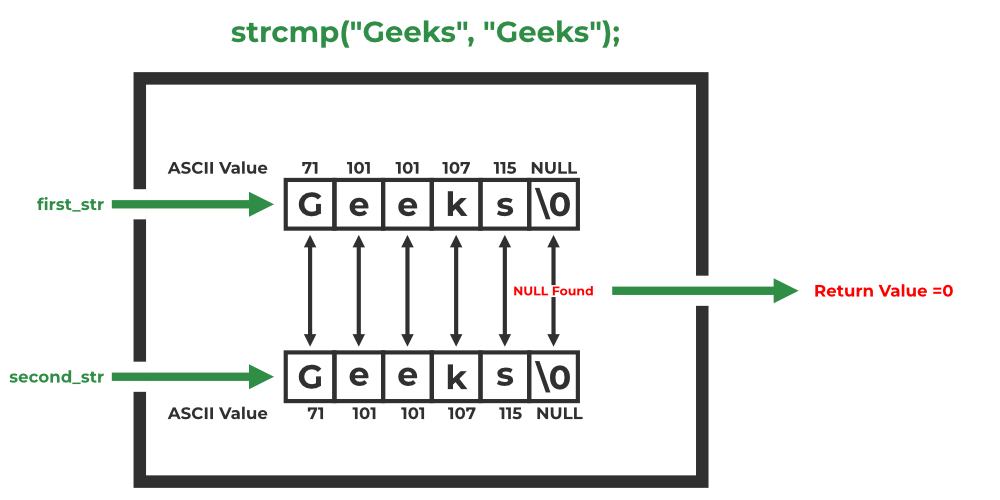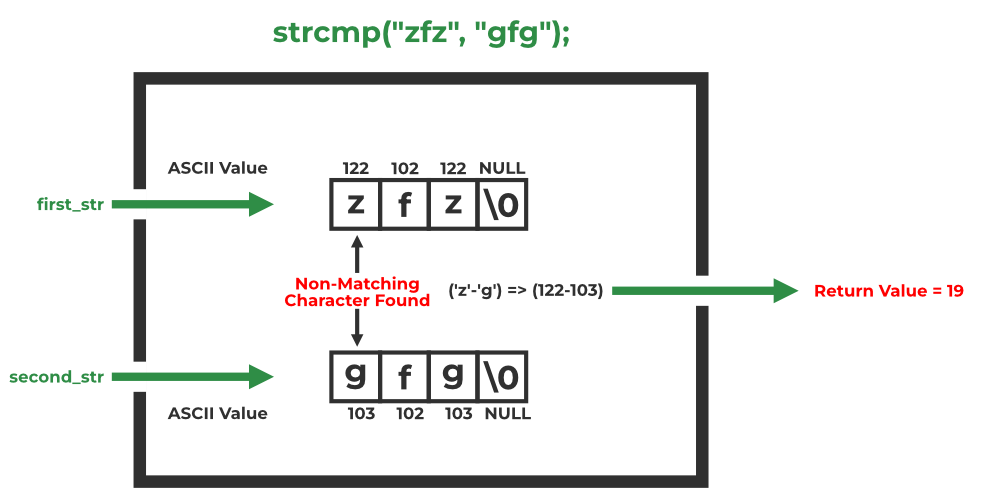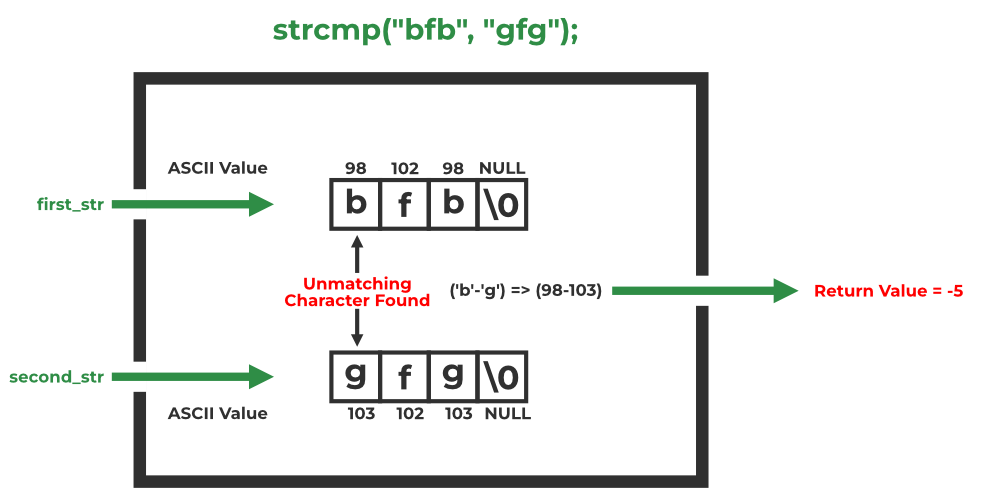# C strcmp()

In C language, the <string.h> header file contains the Standard String Library that contains some useful and commonly used string manipulation functions. In this article, we will see how to compare strings in C using the function strcmp().

## What is strcmp() in C?

C strcmp() is a built-in library function that is used for string comparison. This function takes two strings (array of characters) as arguments, compares these two strings lexicographically, and then returns 0,1, or -1 as the result. It is defined inside <string.h> header file with its prototype as follows:

## Syntax of strcmp() in C

`strcmp(first_str, second_str );`

## Parameters of strcmp() in C

This function takes two strings (array of characters) as parameters:

• first_str: First string is taken as a pointer to the constant character (i.e. immutable string).
• second_str: Second string is taken as a pointer to a constant character.

Note: The reason arguments are taken as const char * instead of only char * is so that the function could not modify the string and also make them applicable for constant strings.

## Return Value of strcmp() in C

The strcmp() function returns three different values after the comparison of the two strings which are as follows:

### 1. Zero ( 0 )

A value equal to zero when both strings are found to be identical. That is, all of the characters in both strings are the same.

### 2. Greater than Zero ( > 0 )

A value greater than zero is returned when the first not-matching character in first_str has a greater ASCII value than the corresponding character in second_str or we can also say that if the character in first_str is lexicographically after the character of second_str, then zero is returned.

### 3. Lesser than Zero ( < 0 )

A value less than zero is returned when the first not-matching character in first_str has a lesser ASCII value than the corresponding character in second_str. We can also say that if the character in first_str is lexicographically before the character of second_str, zero is returned.

## How to use the strcmp() function in C

The following example demonstrates how to use the strcmp() function in C:

## C

 `// C Program to Demonstrate the use of strcmp() function` `#include ` `#include `   `int` `main()` `{` `    ``// declaring two same string` `    ``char``* first_str = ``"Geeks"``;` `    ``char``* second_str = ``"Geeks"``;`   `    ``// printing the strings` `    ``printf``(``"First String: %s\n"``, first_str);` `    ``printf``(``"Second String: %s\n"``, second_str);`   `    ``// printing the return value of the strcmp()` `    ``printf``(``"Return value of strcmp(): %d"``,` `           ``strcmp``(first_str, second_str));`   `    ``return` `0;` `}`

Output

```First String: Geeks
Second String: Geeks
Return value of strcmp(): 0```

## How strcmp() in C works?

C strcmp() function works by comparing the two strings lexicographically. It means that it compares the ASCII value of each character till the non-matching value is found or the NULL character is found. The working of the C strcmp() function can be described as follows:

1. It starts with comparing the ASCII values of the first characters of both strings.

2. If the first characters in both strings are equal, then this function will check the second character, if they are also equal, then it will check the third, and so on till the first unmatched character is found or the NULL character is found.

3. If a NULL character is found, the function returns zero as both strings will be the same.4. If a non-matching character is found,

• If the ASCII value of the character of the first string is greater than that of the second string, then the positive difference ( > 0) between their ASCII values is returned.• If the ASCII value of the character of the first string is less than that of the second string, then the negative difference ( < 0) between their ASCII values is returned.All of these three cases are demonstrated in the below examples.

## Examples of strcmp() in C

### Example 1. strcmp() behavior for identical strings

This program illustrates the behavior of the strcmp() function for identical strings.

## C

 `// C program to illustrate` `// strcmp() function` `#include` `#include`   `int` `main()` `{` `    `  `    ``char` `first_str[] = ``"g f g"``;` `    ``char` `second_str[] = ``"g f g"``;` `    `  `    ``// Using strcmp()` `    ``int` `res = ``strcmp``(first_str, second_str);` `    `  `    ``if` `(res==0)` `        ``printf``(``"Strings are equal"``);` `    ``else` `        ``printf``(``"Strings are unequal"``);` `    `  `    ``printf``(``"\nValue returned by strcmp() is: %d"` `, res);` `    ``return` `0;` `}`

Output

```Strings are equal
Value returned by strcmp() is: 0```

### Example 2. strcmp() behavior for the lexicographically greater first string

The below example demonstrates the strcmp() function behavior for the lexicographically greater first string.

## C

 `// C program to illustrate` `// strcmp() function` `#include` `#include` `int` `main()` `{` `    ``// z has greater ASCII value than g` `    ``char` `first_str[] = ``"zfz"``;` `    ``char` `second_str[] = ``"gfg"``;` `    `  `    ``int` `res = ``strcmp``(first_str, second_str);` `    `  `    ``if` `(res==0)` `        ``printf``(``"Strings are equal"``);` `    ``else` `        ``printf``(``"Strings are unequal"``);` `        `  `    ``printf``(``"\nValue of result: %d"` `, res);` `    `  `    ``return` `0;` `}`

Output

```Strings are unequal
Value of result: 19```

### Example 3. strcmp() behavior for the lexicographically smaller first string.

The below example demonstrates the strcmp() function behavior for the lexicographically smaller first string.

## C

 `// C program to illustrate` `// strcmp() function` `#include` `#include` `int` `main()` `{` `    ``// b has less ASCII value than g` `    ``char` `first_str[] = ``"bfb"``;` `    ``char` `second_str[] = ``"gfg"``;` `    `  `    ``int` `res = ``strcmp``(first_str, second_str);` `    `  `    ``if` `(res==0)` `        ``printf``(``"Strings are equal"``);` `    ``else` `        ``printf``(``"Strings are unequal"``);` `        `  `    ``printf``(``"\nValue returned by strcmp() is: %d"` `, res);` `    `  `    `  `    ``return` `0;` `}`

Output

```Strings are unequal
Value returned by strcmp() is: -5```

## Conclusion

In this article, we discussed the C standard library function strcmp() which is used to compare two strings lexicographically. The standard library contains some useful and frequently used functions that make programming easier as they help to avoid rewriting the commonly used function again and again when needed.

## FAQs on strcmp() in C

### 1. How can we compare two strings in C?

Ans: We can use the strcmp() function which is defined inside <string.h> header file to lexicographically compare two strings (array of characters).

### 2. What is the function prototype of strcmp() in C?

Ans: The function prototype of the strcmp() function is as follows:

`int strcmp(const char* lhs, const char* rhs);`

### 3. When strcmp() function return zero?

Ans: The strcmp() function returns zero when the two strings are identical.

### 4. What does the positive return value by the strcmp() function mean?

Ans: The strcmp() function returns a positive value when the first string is lexicographically greater than the second string.

### 5. What does the negative return value of the strcmp() function mean?

Ans: The negative value return by the strcmp() function means that the first string is lexicographically smaller than the second string.

### 6. How does the strcmp() function compare two strings in C?

Ans: The strcmp() function compares the ASCII values of each character of the two strings till the non-matching character or NULL character is found.

### 7. Can the strcmp() function be used to compare non-string data types in C?

Ans: No, the strcmp() function cannot compare non-string data types in C. It can only compare the mutable or immutable string data type terminating with a NULL character.

### Related Articles:

Whether you're preparing for your first job interview or aiming to upskill in this ever-evolving tech landscape, GeeksforGeeks Courses are your key to success. We provide top-quality content at affordable prices, all geared towards accelerating your growth in a time-bound manner. Join the millions we've already empowered, and we're here to do the same for you. Don't miss out - check it out now!

Previous
Next